# Variable g Pendulum

Mã sản phẩm: P041213

Thương hiệu: AELAB

Liên hệ: 0963.826.083
Mô tả sản phẩm

Investigate the oscillation behaviour of a pendulum (rod pendulum) by varying the magnitude of the components of the acceleration of gravity which are decisive for the oscillation period. The pendulum that is to be used is constructed in such a manner that its oscillation plane can be progressively rotated from a vertical orientation to a horizontal one.

1. Measurement of the oscillation period of the pendulum as a function of the angle of inclination α of the oscillation plane for two different pendulum lengths.

2. Graphical analysis of the measured correlations and a comparison with the theoretical curves, which have been standardised with the measured value at α = 0.

3. Calculation of the effective pendulum length L for the acceleration of gravity, which is assumed to be known. Comparison of this value with the distance between the pivot point of the pendulum and the centre of gravity of the mobile pendulum weight.

4. On the moon’s surface the “lunar acceleration of gravity” gm is only 16.6% of the earth’s acceleration of gravity g. Calculate the angle α and set it on the device such that the pendulum in the laboratory oscillates with the same oscillation period with which it would oscillate on the moon in a perpendicular position. Compare the measured oscillation period with the calculated one.

### Pictures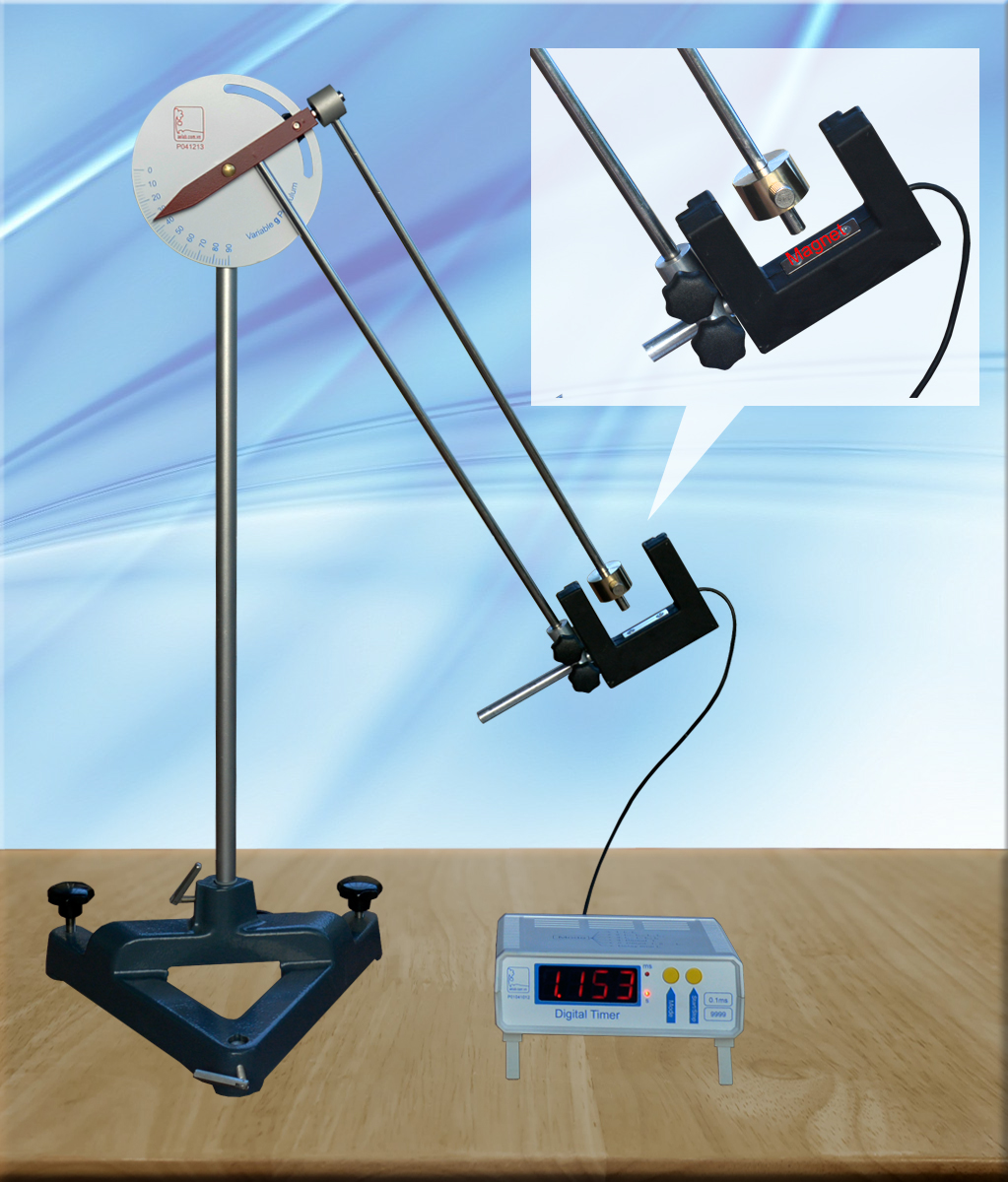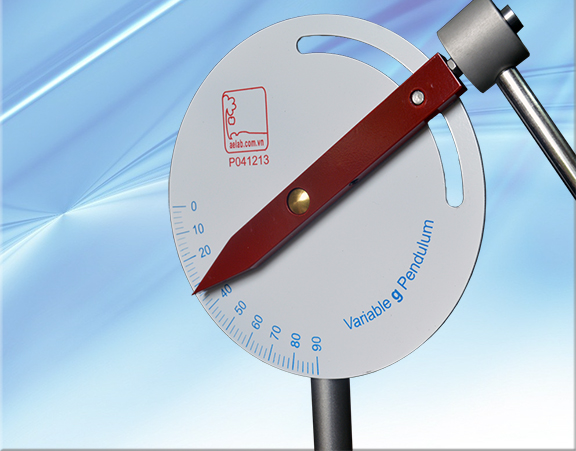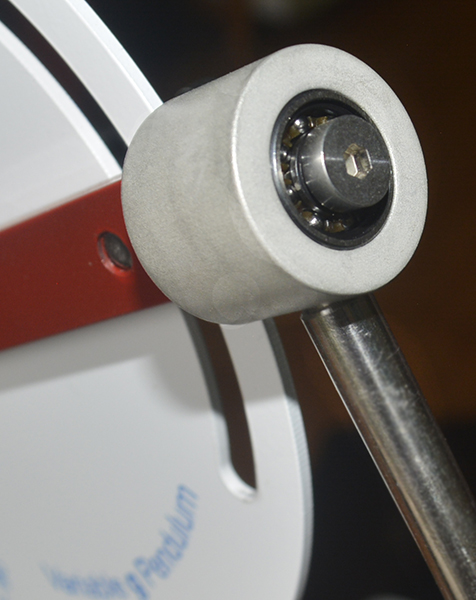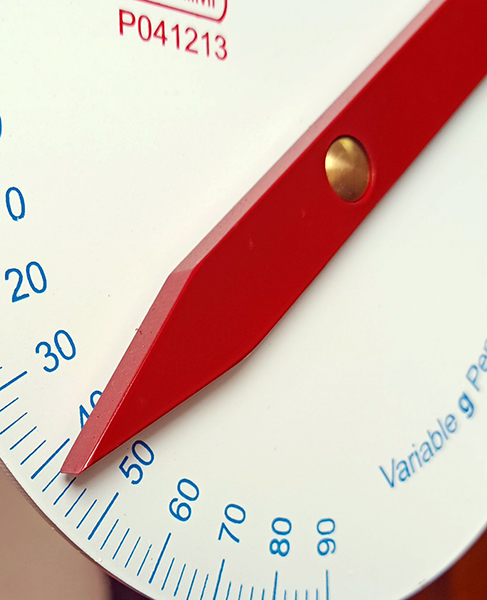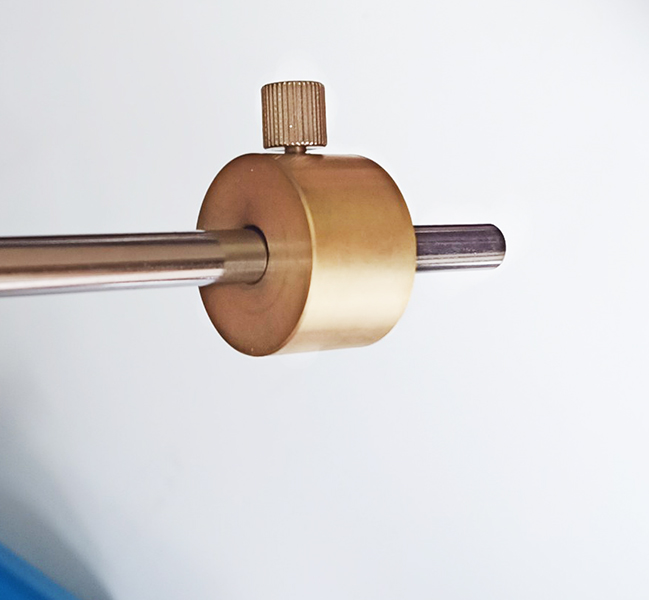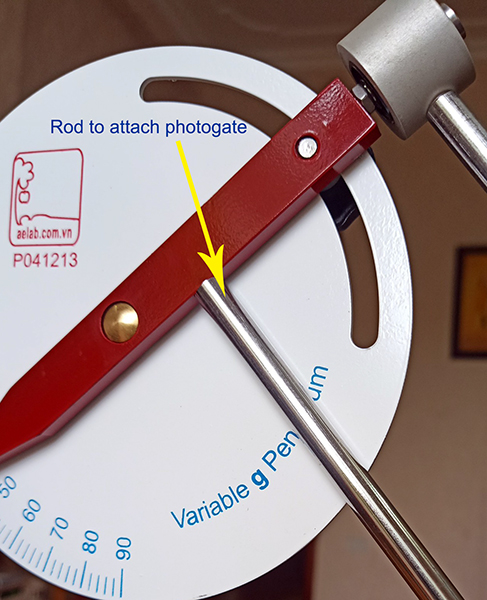### Theory and data

When the axis is tilted, the component of the gravitational acceleration g that is parallel to the axis gpar is rendered ineffective by the fact that the axis is fixed (see fig. below). The
remaining component that is effective geff is given by the following equation: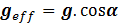(1)

The period of oscillation of the pendulum: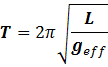(2)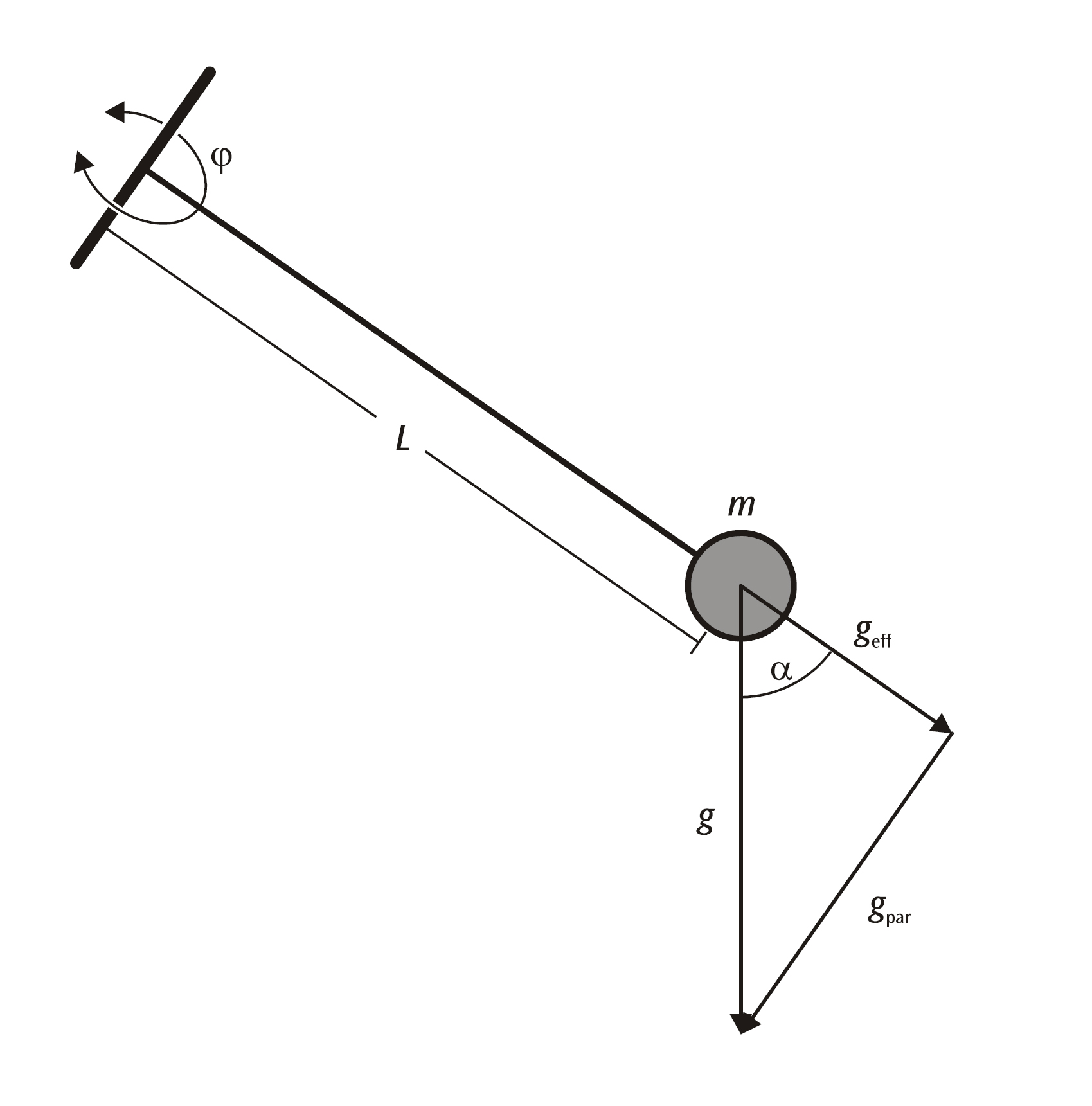Assumption in Hanoi g = 9.81.

With α = 0. Calculation of the effective pendulum length Leff for L = 300mm and L = 445mm.

geff  = g = 9.81

Use formula (2):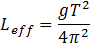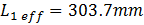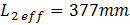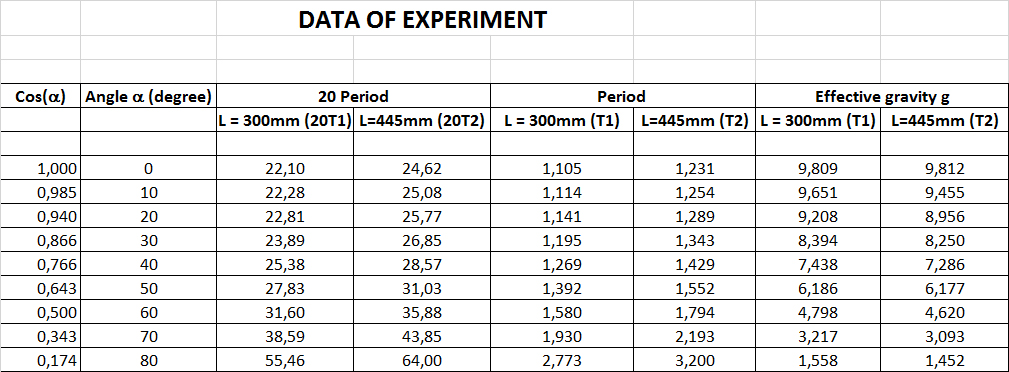Plots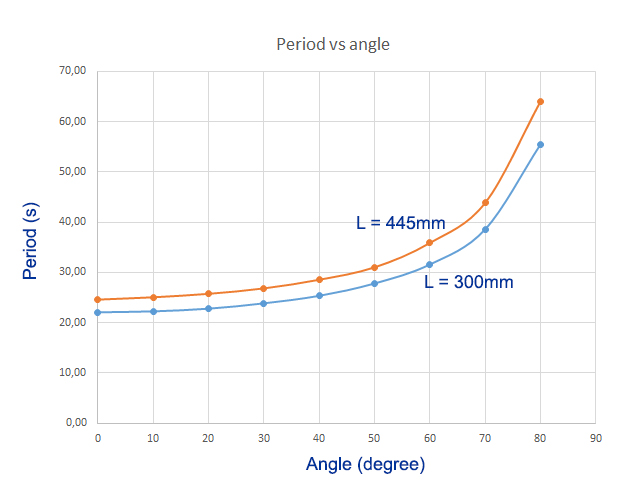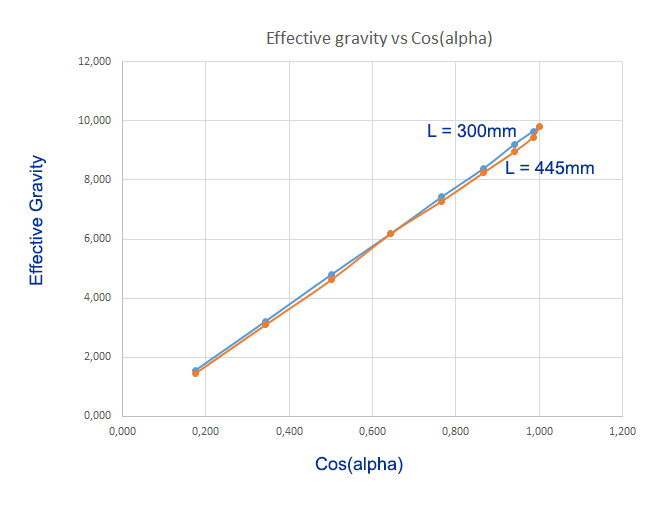gmoon = 16.6%g = 1.625

Based on tables and graphs
We calculate it with a rotation angle of 79 degrees
The pendulum will swing like it is on the moon

### Video

Oscillation on Earth and Moon